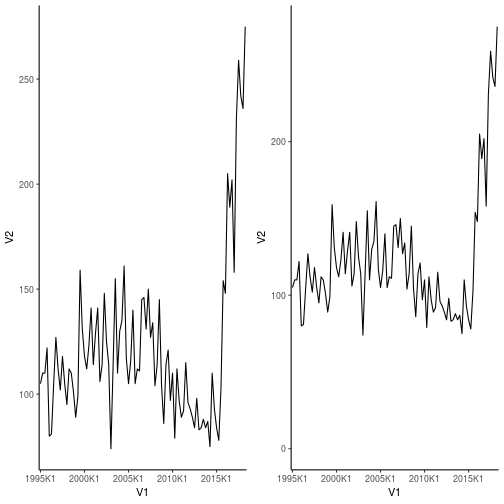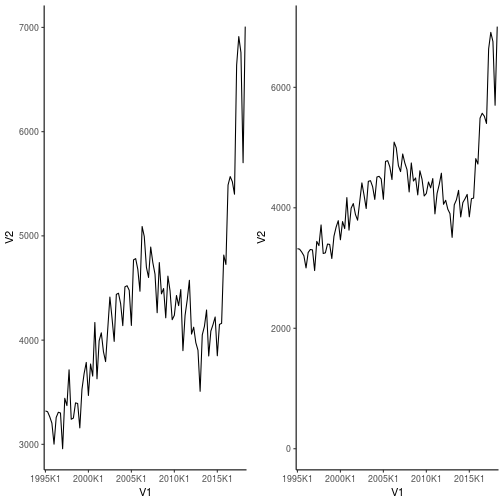# Project Euler 39

We’re looking at Pythagorean triplets, that is equations where a, b and c are integers, and:

a2 + b2 = c2

The triangle defined by a,b,c has a perimeter.

The triplet 20,48,52 fulfills the equation, 202 + 482 = 522. And the perimeter of the triangle is 20 + 48 + 52 = 120

Which perimeter p, smaller than 1000, has the most solutions?

So, we have two equations:

a2 + b2 = c2

p = a + b + c

We can write

c = p – a – b

And substitute that into the first equation:

a2 + b2 = (p – a -b)2

Expanding the paranthesis:

a2 + b2 = p2 – ap – bp – ap + a2 + ab – bp + ab + b2

Cancelling:

0 = p2 – 2ap – 2bp + 2ab

Isolating b:

0 = p2 – 2ap – b(2p – 2a)

b(2p – 2a) = p2 – 2ap

b = (p2 – 2ap)/(2p – 2a)

So. For a given value of p, we can run through all possible values of a and get b. If b is integer, we have a solution that satisfies the constraints.

The smallest value of a we need to check is 1. But what is the largest value of a for a given value of p?

We can see from the pythagorean equation, that a =< b < c. a might be larger than b, but we can then just switch a and b. So it holds. What follows from that, is that a =< p/3.

What else? If a and b are both even, a2 and b2 are also even, then c2 is even, and then c is even, and therefore p = a + b + c is also even.

If a and b are both uneven, a2 and b2 are also uneven, and c2 is then even. c is then even. And therefore p = a + b + c must be even.

If either a or b are uneven, either a2 or b2 is uneven. Then c2 is uneven, and c is then uneven. Therefore p = a + b + c must be even.

So. I only need to check even values of p. That halves the number of values to check.

Allright, time to write some code:

``````current_best_number_of_solutions <- 0

for(p in seq(2,1000,by=2)){
solutions_for_current_p <- 0
for(a in 1:ceiling(p/3)){
if(!(p**2-2*a*p)%%(2*p-2*a)){
solutions_for_current_p <- solutions_for_current_p + 1
}
}
if(solutions_for_current_p > current_best_number_of_solutions){
current_best_p <- p
current_best_number_of_solutions <- solutions_for_current_p
}
}

``````

current_best_number_of_solutions is initialized to 0.

For every p from 2 to 1000, in steps of 2 (only checking even values of p), I set the number of solutions_for_current_p to 0.

For every value a from 1 to p/3 – rounded to to an integer: If !(p2-2*a*p)%%(2*p-2*a) is true, that is, if the remainder of (p2-2*a*p)/(2*p-2*a) is 0, I increment the solutions_for_current_p.

After running through all possible values of a for the value of p we have reached in the for-loop:

If the number of solutions for this value of p is larger, than the previous current_best_number_of_solutions, we have found a value of p that has a higher number of solutions than any previous value of p we have examined. In that case, set the current_best_p to the current value of p. And the current_best_number_of_solutions to the number of solutions we have found for the value of p.

If not, dont change anything, reset solutions_for_current_p and check a new value of p.

# Crimes against graphs

Crime is a bad thing. No doubt about it. And one of the main topics in todays debate climate is – “those ‘orrible immigrants are very criminal. Look at these numbers, they prove it!”. Usually written with caps-lock engaged.

Well. Maybe they are, and maybe they do. But if you want to use statistics to prove it – pretty please, do not obfuscate the numbers.

This is an example. A blog post from one of the more notable danish newspapers. In the US it would be regarded as communist, in the rest of the world we would think of it as relatively conservative.

https://kulturkamp.blogs.berlingske.dk/2018/08/17/anmeldte-voldtaegter-og-voldsforbrydelser-er-paa-det-hoejeste-nogensinde/

The claim is, that the number of reported rapes and other violent crimes in Denmark, are the highest ever. That is because of the increasing numbers of immigrants in Denmark, especially muslims. Use Google translate if you want the details.

Again, that claim might be true. But the graphs in the post, that supposedly documents the claim, are misleading. To say the least.

First – the numbers come from the Danish Statistical Bureau. They have a disclaimer, telling us that changes to the danish penal code, means that a number of sexual offenses have been reclassified as violent crimes since 2013. If the number of violent crimes suddenly includes crimes that did not use to be classified as violent crimes, that number will increase. Not much of a surprise. Yes, the post asks why the numbers are still increasing after that reclassification. One should expect them to level off. And again the post may have a valid point. I don’t know. But what I do know, is that the graphs are misleading.

Heres why. The y-axis has been cut of. Lets recreate the graphs, and take a look.

There are two graphs. The first shows the number of reported cases of rape from 1995 until today.

The second shows the total number of reported cases of violent crimes in the same period. Both sets of data comes from http://www.statistikbanken.dk/.

We’re going to need some libraries:

``````library(ggplot2)
library(gridExtra)
``````

Lets begin by pulling the data.

There might be better ways, but I’ve simply downloaded the data. Two files:

``````violence <- read.csv("tab1.csv", sep=";", skip=3, header=F)
violence <- violence[1:(nrow(violence)-7),]
rape <- rape[1:(nrow(rape)-7),]
``````

The last seven lines are the notes about changes in which cases are counted in this statistics. I think that is a pretty important point, but they are difficult to plot.

The graph for rape, as presented in the post, and with a more sensible y-axis:

``````post <- ggplot(rape, aes(x=V1, y=V2)) +
geom_line(group=1) +
scale_x_discrete(breaks = rape\$V1[seq(1, length(rape\$V1), by = 20)]) +
theme_classic()

nice <- post + ylim(0,max(rape\$V2))
grid.arrange(post, nice, ncol=2)
``````And the one for violent crimes in general, again with the original on the left, and the better on the right:

``````post <- ggplot(violence, aes(x=V1, y=V2)) +
geom_line(group=1) +
scale_x_discrete(breaks = violence\$V1[seq(1, length(violence\$V1), by = 20)]) +
theme_classic()

nice <- post + ylim(0,max(violence\$V2))
grid.arrange(post, nice, ncol=2)
``````So, still, some pretty scary increases. And the change in what is counted should give an increase. But that increase should level off, which it does not. Clearly something is not as it should be. But lets be honest, the graphs on the right are not quite as scary as the ones on the left.

Also – that change in what is counted as sexual assaults – it can explain the initial increase, but then it should level off. That is a fair point. However, there were other things that changed in the period. #metoo for example. I think it would be reasonable to expect that a lot of cases that used to be brushed of as not very important, are now finally being reported. The numbers might actually have leveled off without #metoo.

Anyway, my point is, that if you want to use graphs to support your claims, do NOT cut off the y-axis to make them look more convincing.